# How to add a frame to a seaborn heatmap figure in Python?

• Difficulty Level : Easy
• Last Updated : 03 Jan, 2021

A heatmap is a graphical representation of data where values are depicted by color. They make it easy to understand complex data at a glance. Heatmaps can be easily drawn using seaborn in python. In this article, we are going to add a frame to a seaborn heatmap figure in Python.

Syntax: seaborn.heatmap(data, *, vmin=None, vmax=None, cmap=None, center=None, annot_kws=None, linewidths=0, linecolor=’white’, cbar=True, **kwargs)

Important Parameters:

• data: 2D dataset that can be coerced into an ndarray.
• linewidths: Width of the lines that will divide each cell.
• linecolor: Color of the lines that will divide each cell.
• cbar: Whether to draw a colorbar.

All the parameters except data are optional.

Returns: An object of type matplotlib.axes._subplots.AxesSubplot

### Create a heatmap

To draw the heatmap we will use the in-built data set of seaborn. Seaborn has many in-built data sets like titanic.csv, penguins.csv, flights.csv, exercise.csv. We can also make our data set it should just be a rectangular ndarray.

## Python3

 `# Import libraries``import` `seaborn as sns``import` `matplotlib.pyplot as plt`` ` `# Preparing dataset``example ``=` `sns.load_dataset(``"flights"``)``example ``=` `example.pivot(``"month"``, ``"year"``,``                        ``"passengers"``)`` ` `# Creating plot``res ``=` `sns.heatmap(example)`` ` `# show plot``plt.show()`

Output: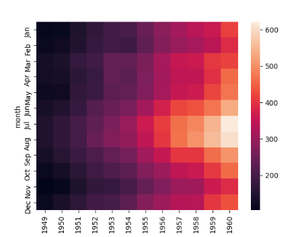basic heatmap

### There are two ways of drawing the frame around a heatmap:

1. Using axhline and axvline.
2. Using spines (more optimal)

Method 1: Using axhline and axvline

The Axes.axhline() and Axes.axvline() function in axes module of matplotlib library is used to add a horizontal and vertical line across the axis respectively.

We can draw two horizontal lines from y=0 and from y= number of rows in our dataset and it will draw a frame covering two sides of our heatmap. Then we can draw two vertical lines from x=0 and x=number of columns in our dataset and it will draw a frame covering the remaining two sides so our heatmap will have a complete frame.

Note: It is not an optimal way to draw a frame as when we increase the line width is does not consider when it is overlapping the heatmap.

Example 1.

## Python3

 `# Import libraries``import` `seaborn as sns``import` `matplotlib.pyplot as plt`` ` `# Preparing dataset``example ``=` `sns.load_dataset(``"flights"``)``example ``=` `example.pivot(``"month"``, ``"year"``,``                        ``"passengers"``)`` ` `# Creating plot``res ``=` `sns.heatmap(example, cmap ``=` `"BuPu"``)`` ` `# Drawing the frame``res.axhline(y ``=` `0``, color``=``'k'``,linewidth ``=` `10``)``res.axhline(y ``=` `example.shape[``1``], color ``=` `'k'``,``            ``linewidth ``=` `10``)`` ` `res.axvline(x ``=` `0``, color ``=` `'k'``,``            ``linewidth ``=` `10``)`` ` `res.axvline(x ``=` `example.shape[``0``], ``            ``color ``=` `'k'``, linewidth ``=` `10``)`` ` `# show plot``plt.show()`

Output: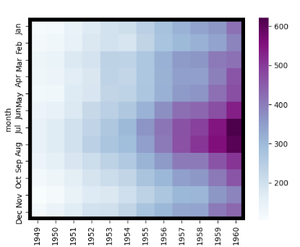Example 2:

## Python3

 `# Import libraries``import` `seaborn as sns``import` `matplotlib.pyplot as plt``import` `numpy as np`` ` `# Preparing dataset``example ``=` `np.random.rand(``10``, ``12``)`` ` `# Creating plot``res ``=` `sns.heatmap(example, cmap ``=` `"magma"``, ``                  ``linewidths ``=` `0.5``)`` ` `# Drawing the frame``res.axhline(y ``=` `0``, color ``=` `'k'``, ``            ``linewidth ``=` `15``)`` ` `res.axhline(y ``=` `10``, color ``=` `'k'``,``            ``linewidth ``=` `15``)`` ` `res.axvline(x ``=` `0``, color ``=` `'k'``,``            ``linewidth ``=` `15``)`` ` `res.axvline(x ``=` `12``, color ``=` `'k'``,``            ``linewidth ``=` `15``)``# show plot``plt.show()`

Output: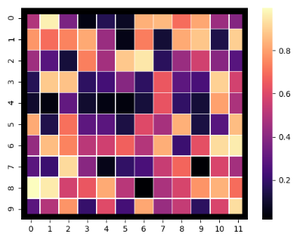Method 2: Using spines

Spines are the lines connecting the axis tick marks and noting the boundaries of the data area. They can be placed at arbitrary positions.

Example 1:

width of the line can be changed using the set_linewidth parameter which accepts a float value as an argument.

## Python3

 `# Import libraries``import` `seaborn as sns``import` `matplotlib.pyplot as plt`` ` `# Preparing dataset``example ``=` `sns.load_dataset(``"flights"``)``example ``=` `example.pivot(``"month"``, ``"year"``, ``                        ``"passengers"``)`` ` `# Creating plot``res ``=` `sns.heatmap(example, cmap ``=` `"Purples"``)`` ` `# Drawing the frame``for` `_, spine ``in` `res.spines.items():``    ``spine.set_visible(``True``)``    ``spine.set_linewidth(``5``)`` ` `# show plot``plt.show()`

Output: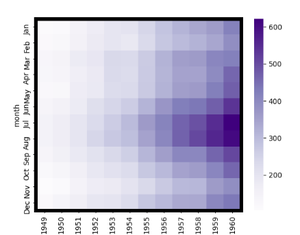Example 2:

We can specify the style of the frame using the set_linestyle parameter of the spine(solid, dashed, dashdot, dotted).

## Python3

 `# Import libraries``import` `seaborn as sns``import` `matplotlib.pyplot as plt``import` `numpy as np`` ` `# Preparing dataset``example ``=` `np.random.rand(``10``, ``12``)`` ` `# Creating plot``res ``=` `sns.heatmap(example, cmap ``=` `"Greens"``,``                  ``linewidths ``=` `2``,``                  ``linecolor ``=` `"white"``)`` ` `# Drawing the frame``for` `_, spine ``in` `res.spines.items():``    ``spine.set_visible(``True``)``    ``spine.set_linewidth(``3``)``    ``spine.set_linestyle(``"dashdot"``)`` ` `# show plot``plt.show()`

Output: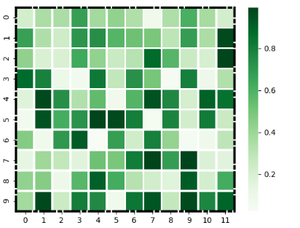My Personal Notes arrow_drop_up